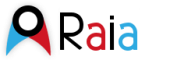## Dataset: Intecmar netCDF Files

• Data format: netCDF
• Data type: GRID
• Naming Authority: Intecmar - Puertos del Estado
• ID: ThreddsIntecmar

### Documentation:

• Summary: Surface ocean velocities estimated from HF-Radar are representative of the upper 0.3 - 2.5 meters of the ocean. The main objective of the near-real time processing is to produce the best product from available data at the time of processing.

### Keywords:

• HF Radar, Galicia, surface sea water velocity, near-real time

### Variables:

• Vocabulary [CF-1.4]:
• latitude (degree_north) = Latitude = latitude
• longitude (degree_east) = Longitude = longitude
• eastward_sea_water_velocity (m s-1) = Surface Eastward Sea Water Velocity = eastward_sea_water_velocity
• northward_sea_water_velocity (m s-1) = Surface Northward Sea Water Velocity = northward_sea_water_velocity
• Vocabulary [No controlled]:
• standard_deviation_u (m s-1) = Standard Deviation U =
• standard_deviation_v (m s-1) = Standard Deviation V =
• covariance (m2 s-2) = Covariance =

### GeospatialCoverage:

• Longitude: -11.4172 to -19.2258 degrees_east
• Latitude: 44.6986 to 86.1026 degrees_north